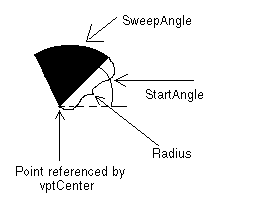﻿ VECTORARC | Used Structures | Vector C API Help
In This Topic ▼

# VECTORARC

````typedef  struct  tagVECTORARC `
`{ `
`   VECTOROBJECT  Object; `
`   VECTORPOINT  Point; `
`   VECTORPEN  Pen; `
`   L_DOUBLE  Radius; `
`   L_DOUBLE  StartAngle; `
`   L_DOUBLE  SweepAngle; `
`}  VECTORARC,  *  pVECTORARC; ````

The VECTORARC structure holds information about a vector arc object.

## Members

Abstract object.

### VECTORPOINT Point

A VECTORPOINT structure that contains the vertex of a vector arc object, about which the arc sweeps.

### VECTORPEN Pen

VECTORPEN structure that contains information about the pen to use when drawing the vector arc object.

### L_DOUBLE Radius

Length of the radius of the vector arc object. This value is in logical units.

### L_DOUBLE StartAngle

The angle at which to begin the vector arc. Values should be between 0 and 360 degrees. Values less than 0 and greater than 360 will be modified internally.

### L_DOUBLE SweepAngle

The angle through which to sweep the vector arc. Values should be between 360 and +360 degrees. Positive angles will be drawn in a counter-clockwise direction from StartAngle. Negative angles will be drawn in a clockwise direction.

## Comments

nSize of the Object member must be the sizeof this structure.

nType of the Object member must be VECTOR_ARC.

pVECTORARC is a pointer to a VECTORARC structure.

The diagram below gives a graphical representation of the Point, Radius, StartAngle and SweepAngle parameters.Help Version 21.0.2021.4.7
Products | Support | Contact Us | Intellectual Property Notices
© 1991-2021 LEAD Technologies, Inc. All Rights Reserved.